# Derivative

One of the basic concepts in mathematical analysis. Suppose that a real-valued functionof a real variableis defined in a neighbourhood of a pointand that there exists a finite or infinite limit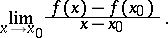(*)

This limit is called the derivative of the functionat the point. If one sets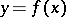,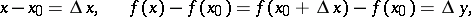then the limit (*) can be written as:Also the notations,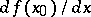,,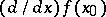, and some others are used to denote this limit.

The operation of computing the derivative is called differentiation. If the derivative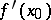is finite,is said to be differentiable at the point. A function differentiable at every point of a set is said to be differentiable on that set. A differentiable function is always continuous. However, there are continuous functions that have no derivative at any point of a given interval (see Non-differentiable function).

Let a functionbe differentiable in an interval. Its derivative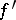may turn out to be a discontinuous function. However, according to Baire's classification (see Baire classes) it is always a function of the first class and has the Darboux property: If it takes two values, it takes every intermediate value as well.

A generalization of the concept of the derivative is the concept of a derivative over a set. Suppose that a real-valued functionis defined on a setof real numbers, thatis a limit point of, that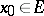, and that there exists a finite or infinite limitThis limit is called the derivative ofover the setat the pointand is denoted by the symbol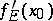. The derivative of a function over a set is a generalization of the concept of a derivative. Variations of the generalization are the concept of a one-sided derivative, a Dini derivative, and an approximate derivative.

The above definition of the derivative (and its generalizations), as well as simple properties of it, extend almost without change to complex-valued and vector-valued functions of a real or complex variable. Moreover, there exists a concept of a derivative of a scalar-valued point function in a Euclidean space(see Gradient), and of a derivative of a set function with respect to a measure (in particular, with respect to area, volume, etc.). The concept of a derivative is extended to vector-valued point functions in an abstract space (see Differentiation of a mapping).

For a geometric and mechanical interpretation of the derivative, the simplest rules of differentiation, higher derivatives, partial derivatives, and also for references see Differential calculus.

G. Choquet has proved that a functiononis of the first Baire class and has the Darboux property (if and) only if there exists a differentiable functiononand a homeomorphismofsuch that. See [a1] for details and references.